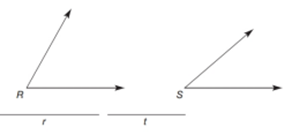Chapter 3.4, Problem 14E### Elementary Geometry for College St...

6th Edition
Daniel C. Alexander + 1 other
ISBN: 9781285195698

#### Solutions

Chapter
Section### Elementary Geometry for College St...

6th Edition
Daniel C. Alexander + 1 other
ISBN: 9781285195698
Textbook Problem
1 views

# In Exercises 13 and 14. use the angles and lengths of sides provided to construct the triangle described. Construct the triangle that has sides of lengths t included by angle R and S .Exercises 13, 14

To determine

To construct:

The triangle with side of length t included by angles R and S.

Explanation

Given:

The given angles are,

Figure (1)

Construction:

Draw a circle of radius t with center at R and name the intersection points.

Figure (2)

Draw two circles of equal radii with center at S and H name the intersection points.

Figure (3)

Draw a circle with radius KL with center M and name the intersection points.

Figure (4)

Draw a ray HN

### Still sussing out bartleby?

Check out a sample textbook solution.

See a sample solution

#### The Solution to Your Study Problems

Bartleby provides explanations to thousands of textbook problems written by our experts, many with advanced degrees!

Get Started

#### Explain what happens during each of the two stages of the two-factor ANOVA.

Essentials of Statistics for The Behavioral Sciences (MindTap Course List)

#### In Exercises 516, evaluate the given quantity. log1/212

Finite Mathematics and Applied Calculus (MindTap Course List)

#### Subtract the following numbers. 18.

Contemporary Mathematics for Business & Consumers

#### The directrix of the conic given by is:

Study Guide for Stewart's Multivariable Calculus, 8th